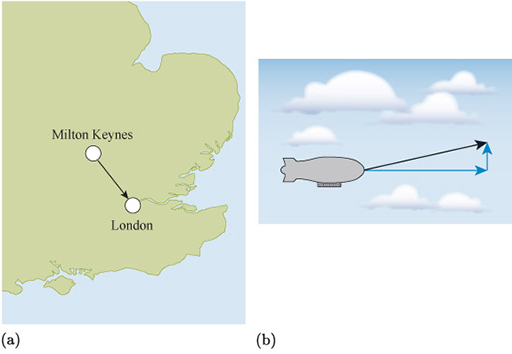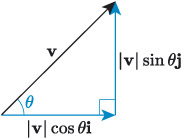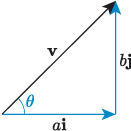Science, Maths & Technology

### Become an OU studentIntroducing vectors for engineering applications

Start this free course now. Just create an account and sign in. Enrol and complete the course for a free statement of participation or digital badge if available.

# 2.3 Converting between vector forms

It is useful to be able to convert between the different forms of vectors, so that we can make use of the form that is most appropriate for a given situation. For example, the vector in Figure 18(a) represents the displacement of London from Milton Keynes, and direction and angle are the most intuitive description for this vector. London is approximately 65﻿ ﻿km south-east of Milton Keynes. Similarly, the vector in Figure 18(b) represents the motion of an aircraft, and for describing the ground speed of the aircraft, a description of the vector in terms of horizontal and vertical components is required.Figure 18 Examples of vectors: (a) displacement of London from Milton Keynes; (b) motion of an aircraft

In both situations it is useful to be able to convert between vector forms. For example, it may be necessary to describe how far east London is from Milton Keynes, and how far south. To describe the direction in which the aircraft is flying, it is useful to use the resultant velocity.

The mathematics that allows us to convert between different forms of vectors.Figure 19 A vector and its components

We have already identified how to calculate the magnitude of the component vectors of a vector. For example, the vector in Figure 19 has magnitude and direction , and its components are defined as and .

Putting these into component form we have the following result.

## Component form of a vector in terms of its magnitude and angle with the positive x-axis

If the vector makes an angle with the positive -axis, then

## Activity 10

Find the component forms of the following vectors. Give your answers to two decimal places.

• a.Vector with magnitude 78 and direction given by an angle of 216° with the positive -axis.

• b.vector with magnitude 4.4 and direction given by an angle of radians with the positive -axis.Figure 20 A vector

Going the other way, to find the magnitude and direction of a vector from its component form, the reasoning is the same as how to convert between Cartesian and polar coordinates. For example, the vector illustrated in Figure 20 has horizontal component of magnitude and vertical component of magnitude , and in component form is given by . To calculate the magnitude of , we can use Pythagoras’ theorem, and to calculate the angle , we can use the inverse tangent function.

## Magnitude and direction of a vector in terms of its components

If the vector has the component form , then its magnitude is given by

and its direction is given by the angle measured anticlockwise from the positive -axis, where

Remember, when using the inverse tan function, care must be taken to ensure that the answer given by a calculator is correct.

## Activity 11

Find the magnitude to two decimal places and direction to one decimal place of the following vectors.

• a.

• b.

• c.